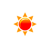# Miles to Kilometer Converter (mi to km)

 Example: 1 mile = 1.609344 km miles: miles km: km
You may also interested in: km to miles Converter

The online miles to km Converter is used to convert the length from miles to kilometers.

#### The miles to km Conversion Formula

You can use the following formula to convert from miles to km :

X(km) = y(miles) × 1.609344

Example: How to convert 10 miles to km

X(km) = 10(miles) × 1.609344

Anwser: 16.09344 km

#### Miles to Kilometers conversion table


Miles (miles) Kilometers (km)
1 miles 1.609344 km
2 miles 3.218688 km
3 miles 4.828032 km
4 miles 6.437376 km
5 miles 8.04672 km
6 miles 9.656064 km
7 miles 11.265408 km
8 miles 12.874752 km
9 miles 14.484096 km
10 miles 16.09344 km
20 miles 32.18688 km
30 miles 48.28032 km
40 miles 64.37376 km
50 miles 80.4672 km
60 miles 96.56064 km
70 miles 112.65408 km
80 miles 128.74752 km
90 miles 144.84096 km
100 miles 160.9344 km
500 miles 804.672 km
1000 miles 1609.344 km
Full miles to km conversion table

#### Frequently asked questions to convert cm to feet

What is the value of 1 mile in km ?

What is the value of 2 mile in km ?

What is the value of 3 mile in km ?

What is the value of 4 mile in km ?

What is the value of 5 mile in km ?

What is the value of 6 mile in km ?

What is the value of 7 mile in km ?

What is the value of 8 mile in km ?

What is the value of 9 mile in km ?

What is the value of 10 mile in km ?

#### Online Converter to convert mile to km

We provide the online converter for free. You can use the mile to km converter to convert miles to km.

#### References

More references for Mile and Kilometre

#### Local Weather Status

Ashburn, US
11th November, 2019 Monday
Clear Sky17.78 - 21
Humidity: 34 %
Wind: 4.1 km/h